8.2 Graphs of the other trigonometric functions  (Page 9/9)

 Page 9 / 9

How can the graph of $\text{\hspace{0.17em}}y=\mathrm{cos}\text{\hspace{0.17em}}x\text{\hspace{0.17em}}$ be used to construct the graph of $\text{\hspace{0.17em}}y=\mathrm{sec}\text{\hspace{0.17em}}x?$

Explain why the period of $\text{\hspace{0.17em}}\mathrm{tan}\text{\hspace{0.17em}}x\text{\hspace{0.17em}}$ is equal to $\text{\hspace{0.17em}}\pi .$

Answers will vary. Using the unit circle, one can show that $\text{\hspace{0.17em}}\mathrm{tan}\left(x+\pi \right)=\mathrm{tan}\text{\hspace{0.17em}}x.\text{\hspace{0.17em}}$

Why are there no intercepts on the graph of $\text{\hspace{0.17em}}y=\mathrm{csc}\text{\hspace{0.17em}}x?$

How does the period of $\text{\hspace{0.17em}}y=\mathrm{csc}\text{\hspace{0.17em}}x\text{\hspace{0.17em}}$ compare with the period of $\text{\hspace{0.17em}}y=\mathrm{sin}\text{\hspace{0.17em}}x?$

The period is the same: $\text{\hspace{0.17em}}2\pi .$

Algebraic

For the following exercises, match each trigonometric function with one of the following graphs.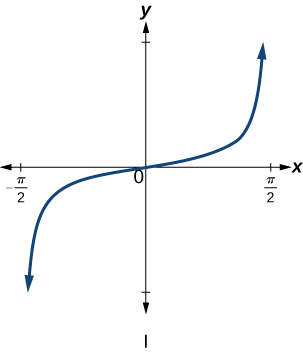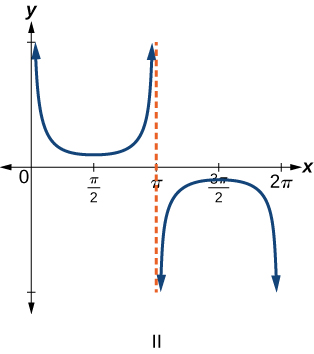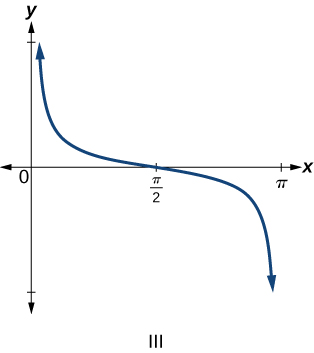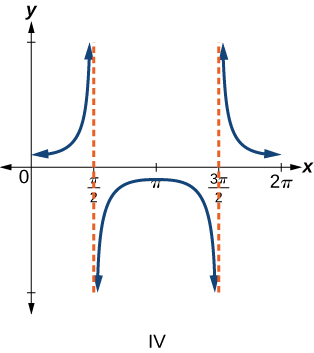$f\left(x\right)=\mathrm{tan}\text{\hspace{0.17em}}x$

$f\left(x\right)=\mathrm{sec}\text{\hspace{0.17em}}x$

IV

$f\left(x\right)=\mathrm{csc}\text{\hspace{0.17em}}x$

$f\left(x\right)=\mathrm{cot}\text{\hspace{0.17em}}x$

III

For the following exercises, find the period and horizontal shift of each of the functions.

$f\left(x\right)=2\mathrm{tan}\left(4x-32\right)$

$h\left(x\right)=2\mathrm{sec}\left(\frac{\pi }{4}\left(x+1\right)\right)$

period: 8; horizontal shift: 1 unit to left

$m\left(x\right)=6\mathrm{csc}\left(\frac{\pi }{3}x+\pi \right)$

If $\text{\hspace{0.17em}}\mathrm{tan}\text{\hspace{0.17em}}x=-1.5,\text{\hspace{0.17em}}$ find $\text{\hspace{0.17em}}\mathrm{tan}\left(-x\right).$

1.5

If $\text{\hspace{0.17em}}\mathrm{sec}\text{\hspace{0.17em}}x=2,\text{\hspace{0.17em}}$ find $\text{\hspace{0.17em}}\mathrm{sec}\left(-x\right).$

If $\text{\hspace{0.17em}}\mathrm{csc}\text{\hspace{0.17em}}x=-5,\text{\hspace{0.17em}}$ find $\text{\hspace{0.17em}}\mathrm{csc}\left(-x\right).$

5

If $\text{\hspace{0.17em}}x\mathrm{sin}\text{\hspace{0.17em}}x=2,\text{\hspace{0.17em}}$ find $\text{\hspace{0.17em}}\left(-x\right)\mathrm{sin}\left(-x\right).$

For the following exercises, rewrite each expression such that the argument $\text{\hspace{0.17em}}x\text{\hspace{0.17em}}$ is positive.

$\mathrm{cot}\left(-x\right)\mathrm{cos}\left(-x\right)+\mathrm{sin}\left(-x\right)$

$-\mathrm{cot}x\mathrm{cos}x-\mathrm{sin}x$

$\mathrm{cos}\left(-x\right)+\mathrm{tan}\left(-x\right)\mathrm{sin}\left(-x\right)$

Graphical

For the following exercises, sketch two periods of the graph for each of the following functions. Identify the stretching factor, period, and asymptotes.

$f\left(x\right)=2\mathrm{tan}\left(4x-32\right)$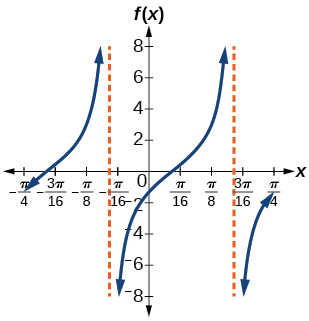stretching factor: 2; period: asymptotes:

$\text{\hspace{0.17em}}h\left(x\right)=2\mathrm{sec}\left(\frac{\pi }{4}\left(x+1\right)\right)\text{\hspace{0.17em}}$

$m\left(x\right)=6\mathrm{csc}\left(\frac{\pi }{3}x+\pi \right)$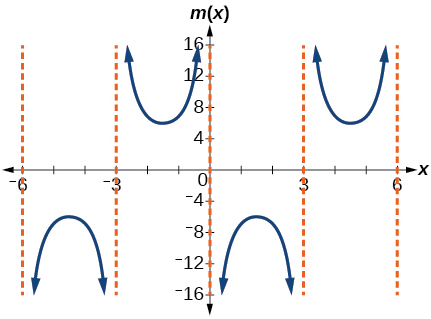stretching factor: 6; period: 6; asymptotes:

$j\left(x\right)=\mathrm{tan}\left(\frac{\pi }{2}x\right)$

$p\left(x\right)=\mathrm{tan}\left(x-\frac{\pi }{2}\right)$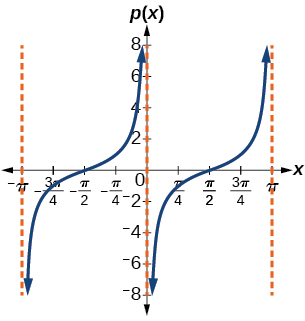stretching factor: 1; period: asymptotes:

$f\left(x\right)=4\mathrm{tan}\left(x\right)$

$f\left(x\right)=\mathrm{tan}\left(x+\frac{\pi }{4}\right)$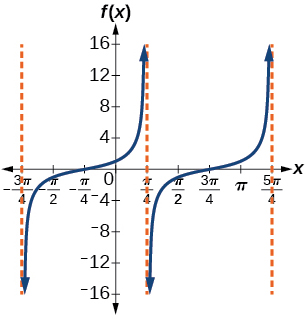Stretching factor: 1; period: asymptotes:

$f\left(x\right)=\pi \mathrm{tan}\left(\pi x-\pi \right)-\pi$

$f\left(x\right)=2\mathrm{csc}\left(x\right)$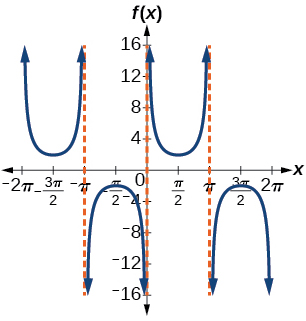stretching factor: 2; period: asymptotes:

$f\left(x\right)=-\frac{1}{4}\mathrm{csc}\left(x\right)$

$f\left(x\right)=4\mathrm{sec}\left(3x\right)$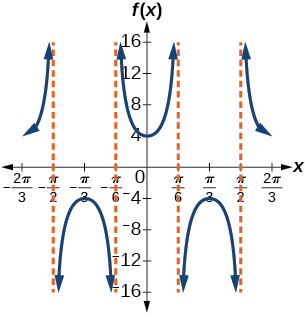stretching factor: 4; period: asymptotes:

$f\left(x\right)=-3\mathrm{cot}\left(2x\right)$

$f\left(x\right)=7\mathrm{sec}\left(5x\right)$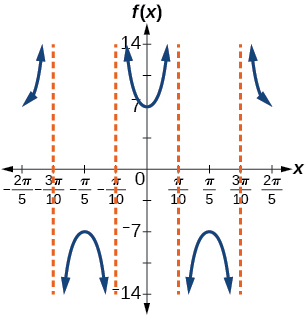stretching factor: 7; period: asymptotes:

$f\left(x\right)=\frac{9}{10}\mathrm{csc}\left(\pi x\right)$

$f\left(x\right)=2\mathrm{csc}\left(x+\frac{\pi }{4}\right)-1$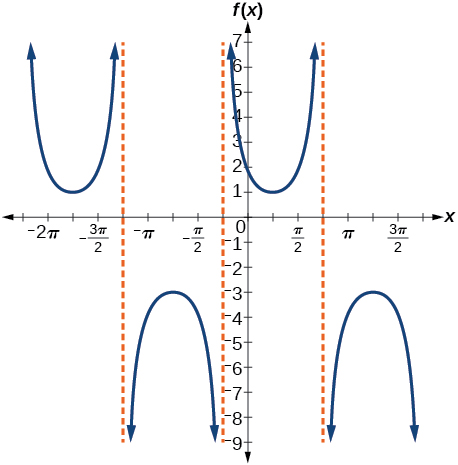stretching factor: 2; period: asymptotes:

$f\left(x\right)=-\mathrm{sec}\left(x-\frac{\pi }{3}\right)-2$

$f\left(x\right)=\frac{7}{5}\mathrm{csc}\left(x-\frac{\pi }{4}\right)$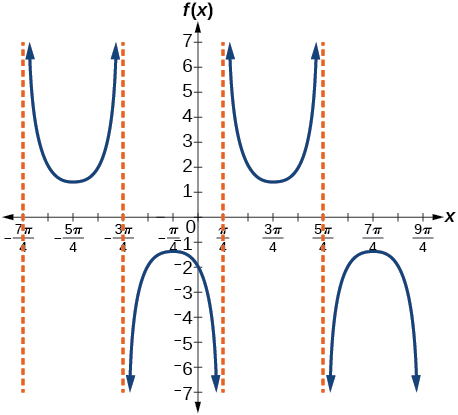stretching factor: period: asymptotes:

$f\left(x\right)=5\left(\mathrm{cot}\left(x+\frac{\pi }{2}\right)-3\right)$

For the following exercises, find and graph two periods of the periodic function with the given stretching factor, $\text{\hspace{0.17em}}|A|,\text{\hspace{0.17em}}$ period, and phase shift.

A tangent curve, $\text{\hspace{0.17em}}A=1,\text{\hspace{0.17em}}$ period of $\text{\hspace{0.17em}}\frac{\pi }{3};\text{\hspace{0.17em}}$ and phase shift $\text{\hspace{0.17em}}\left(h,\text{\hspace{0.17em}}k\right)=\left(\frac{\pi }{4},2\right)$

$y=\mathrm{tan}\left(3\left(x-\frac{\pi }{4}\right)\right)+2$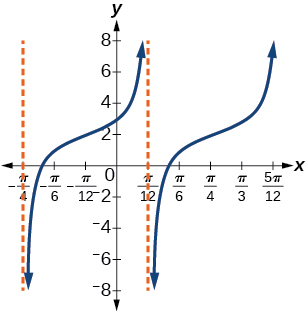A tangent curve, $\text{\hspace{0.17em}}A=-2,\text{\hspace{0.17em}}$ period of $\text{\hspace{0.17em}}\frac{\pi }{4},\text{\hspace{0.17em}}$ and phase shift $\text{\hspace{0.17em}}\left(h,\text{\hspace{0.17em}}k\right)=\left(-\frac{\pi }{4},\text{\hspace{0.17em}}-2\right)$

For the following exercises, find an equation for the graph of each function.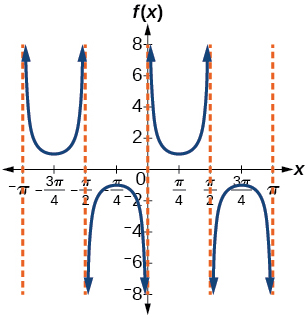$f\left(x\right)=\mathrm{csc}\left(2x\right)$$f\left(x\right)=\mathrm{csc}\left(4x\right)$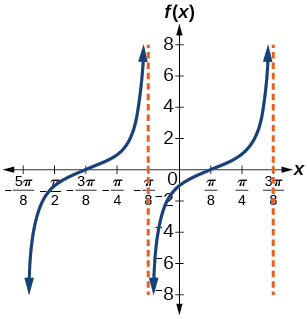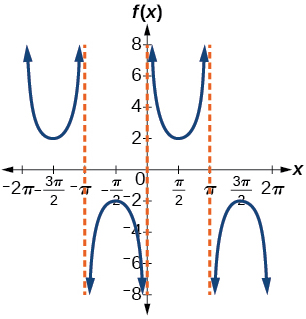$f\left(x\right)=2\mathrm{csc}x$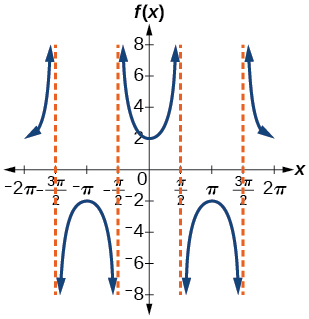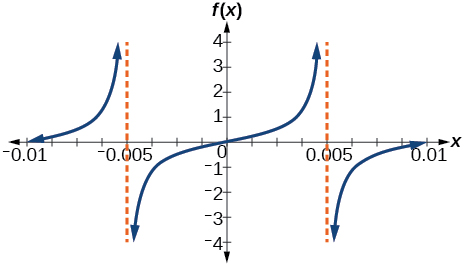$f\left(x\right)=\frac{1}{2}\mathrm{tan}\left(100\pi x\right)$

Technology

For the following exercises, use a graphing calculator to graph two periods of the given function. Note: most graphing calculators do not have a cosecant button; therefore, you will need to input $\text{\hspace{0.17em}}\mathrm{csc}\text{\hspace{0.17em}}x\text{\hspace{0.17em}}$ as $\text{\hspace{0.17em}}\frac{1}{\mathrm{sin}\text{\hspace{0.17em}}x}.$

$f\left(x\right)=|\mathrm{csc}\left(x\right)|$

$f\left(x\right)=|\mathrm{cot}\left(x\right)|$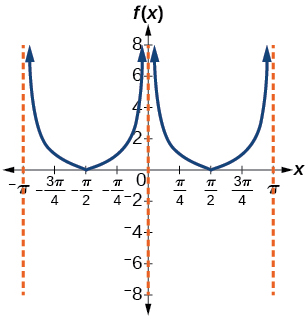$f\left(x\right)={2}^{\mathrm{csc}\left(x\right)}$

$f\left(x\right)=\frac{\mathrm{csc}\left(x\right)}{\mathrm{sec}\left(x\right)}$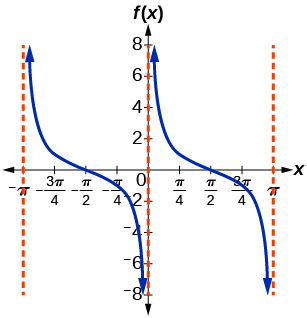Graph $\text{\hspace{0.17em}}f\left(x\right)=1+{\mathrm{sec}}^{2}\left(x\right)-{\mathrm{tan}}^{2}\left(x\right).\text{\hspace{0.17em}}$ What is the function shown in the graph?

$f\left(x\right)=\mathrm{sec}\left(0.001x\right)$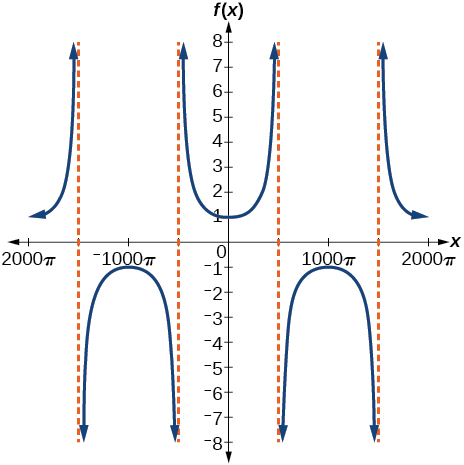$f\left(x\right)=\mathrm{cot}\left(100\pi x\right)$

$f\left(x\right)={\mathrm{sin}}^{2}x+{\mathrm{cos}}^{2}x$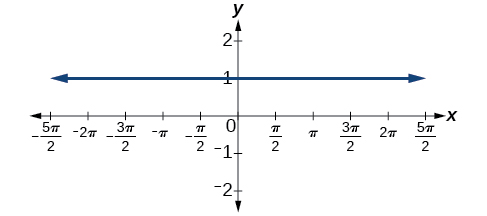Real-world applications

The function $\text{\hspace{0.17em}}f\left(x\right)=20\mathrm{tan}\left(\frac{\pi }{10}x\right)\text{\hspace{0.17em}}$ marks the distance in the movement of a light beam from a police car across a wall for time $\text{\hspace{0.17em}}x,\text{\hspace{0.17em}}$ in seconds, and distance $\text{\hspace{0.17em}}f\left(x\right),$ in feet.

1. Graph on the interval $\text{\hspace{0.17em}}\left[0,\text{\hspace{0.17em}}5\right].$
2. Find and interpret the stretching factor, period, and asymptote.
3. Evaluate $\text{\hspace{0.17em}}f\left(1\right)\text{\hspace{0.17em}}$ and $\text{\hspace{0.17em}}f\left(2.5\right)\text{\hspace{0.17em}}$ and discuss the function’s values at those inputs.

Standing on the shore of a lake, a fisherman sights a boat far in the distance to his left. Let $\text{\hspace{0.17em}}x,\text{\hspace{0.17em}}$ measured in radians, be the angle formed by the line of sight to the ship and a line due north from his position. Assume due north is 0 and $\text{\hspace{0.17em}}x\text{\hspace{0.17em}}$ is measured negative to the left and positive to the right. (See [link] .) The boat travels from due west to due east and, ignoring the curvature of the Earth, the distance $\text{\hspace{0.17em}}d\left(x\right),\text{\hspace{0.17em}}$ in kilometers, from the fisherman to the boat is given by the function $\text{\hspace{0.17em}}d\left(x\right)=1.5\mathrm{sec}\left(x\right).$

1. What is a reasonable domain for $\text{\hspace{0.17em}}d\left(x\right)?$
2. Graph $\text{\hspace{0.17em}}d\left(x\right)\text{\hspace{0.17em}}$ on this domain.
3. Find and discuss the meaning of any vertical asymptotes on the graph of $\text{\hspace{0.17em}}d\left(x\right).$
4. Calculate and interpret $\text{\hspace{0.17em}}d\left(-\frac{\pi }{3}\right).\text{\hspace{0.17em}}$ Round to the second decimal place.
5. Calculate and interpret $\text{\hspace{0.17em}}d\left(\frac{\pi }{6}\right).\text{\hspace{0.17em}}$ Round to the second decimal place.
6. What is the minimum distance between the fisherman and the boat? When does this occur?
1. $\text{\hspace{0.17em}}\left(-\frac{\pi }{2},\text{\hspace{0.17em}}\frac{\pi }{2}\right);\text{\hspace{0.17em}}$
2.3. $\text{\hspace{0.17em}}x=-\frac{\pi }{2}\text{\hspace{0.17em}}$ and $\text{\hspace{0.17em}}x=\frac{\pi }{2};\text{\hspace{0.17em}}$ the distance grows without bound as $\text{\hspace{0.17em}}|x|$ approaches $\text{\hspace{0.17em}}\frac{\pi }{2}\text{\hspace{0.17em}}$ —i.e., at right angles to the line representing due north, the boat would be so far away, the fisherman could not see it;
4. 3; when $\text{\hspace{0.17em}}x=-\frac{\pi }{3},\text{\hspace{0.17em}}$ the boat is 3 km away;
5. 1.73; when $\text{\hspace{0.17em}}x=\frac{\pi }{6},\text{\hspace{0.17em}}$ the boat is about 1.73 km away;
6. 1.5 km; when $\text{\hspace{0.17em}}x=0\text{\hspace{0.17em}}$

A laser rangefinder is locked on a comet approaching Earth. The distance $\text{\hspace{0.17em}}g\left(x\right),\text{\hspace{0.17em}}$ in kilometers, of the comet after $\text{\hspace{0.17em}}x\text{\hspace{0.17em}}$ days, for $\text{\hspace{0.17em}}x\text{\hspace{0.17em}}$ in the interval 0 to 30 days, is given by $\text{\hspace{0.17em}}g\left(x\right)=250,000\mathrm{csc}\left(\frac{\pi }{30}x\right).$

1. Graph $\text{\hspace{0.17em}}g\left(x\right)\text{\hspace{0.17em}}$ on the interval $\text{\hspace{0.17em}}\left[0,\text{\hspace{0.17em}}35\right].$
2. Evaluate $\text{\hspace{0.17em}}g\left(5\right)\text{\hspace{0.17em}}$ and interpret the information.
3. What is the minimum distance between the comet and Earth? When does this occur? To which constant in the equation does this correspond?
4. Find and discuss the meaning of any vertical asymptotes.

A video camera is focused on a rocket on a launching pad 2 miles from the camera. The angle of elevation from the ground to the rocket after $\text{\hspace{0.17em}}x\text{\hspace{0.17em}}$ seconds is $\text{\hspace{0.17em}}\frac{\pi }{120}x.$

1. Write a function expressing the altitude $\text{\hspace{0.17em}}h\left(x\right),\text{\hspace{0.17em}}$ in miles, of the rocket above the ground after $\text{\hspace{0.17em}}x\text{\hspace{0.17em}}$ seconds. Ignore the curvature of the Earth.
2. Graph $\text{\hspace{0.17em}}h\left(x\right)\text{\hspace{0.17em}}$ on the interval $\text{\hspace{0.17em}}\left(0,\text{\hspace{0.17em}}60\right).$
3. Evaluate and interpret the values $\text{\hspace{0.17em}}h\left(0\right)\text{\hspace{0.17em}}$ and $\text{\hspace{0.17em}}h\left(30\right).$
4. What happens to the values of $\text{\hspace{0.17em}}h\left(x\right)\text{\hspace{0.17em}}$ as $\text{\hspace{0.17em}}x\text{\hspace{0.17em}}$ approaches 60 seconds? Interpret the meaning of this in terms of the problem.
1. $h\left(x\right)=2\mathrm{tan}\left(\frac{\pi }{120}x\right);$
2.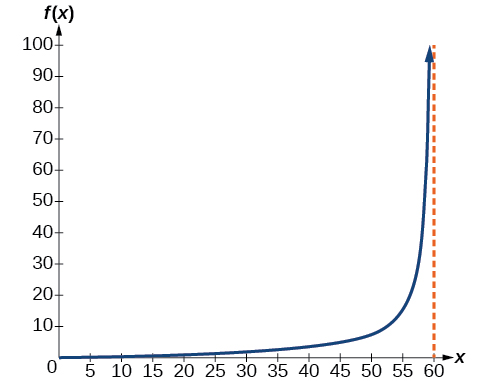3. $h\left(0\right)=0:\text{\hspace{0.17em}}$ after 0 seconds, the rocket is 0 mi above the ground; $h\left(30\right)=2:\text{\hspace{0.17em}}$ after 30 seconds, the rockets is 2 mi high;
4. As $\text{\hspace{0.17em}}x\text{\hspace{0.17em}}$ approaches 60 seconds, the values of $\text{\hspace{0.17em}}h\left(x\right)\text{\hspace{0.17em}}$ grow increasingly large. The distance to the rocket is growing so large that the camera can no longer track it.

A laser rangefinder is locked on a comet approaching Earth. The distance g(x), in kilometers, of the comet after x days, for x in the interval 0 to 30 days, is given by g(x)=250,000csc(π30x). Graph g(x) on the interval [0, 35]. Evaluate g(5)  and interpret the information. What is the minimum distance between the comet and Earth? When does this occur? To which constant in the equation does this correspond? Find and discuss the meaning of any vertical asymptotes.
The sequence is {1,-1,1-1.....} has
how can we solve this problem
Sin(A+B) = sinBcosA+cosBsinA
Prove it
Eseka
Eseka
hi
Joel
June needs 45 gallons of punch. 2 different coolers. Bigger cooler is 5 times as large as smaller cooler. How many gallons in each cooler?
7.5 and 37.5
Nando
find the sum of 28th term of the AP 3+10+17+---------
I think you should say "28 terms" instead of "28th term"
Vedant
the 28th term is 175
Nando
192
Kenneth
if sequence sn is a such that sn>0 for all n and lim sn=0than prove that lim (s1 s2............ sn) ke hole power n =n
write down the polynomial function with root 1/3,2,-3 with solution
if A and B are subspaces of V prove that (A+B)/B=A/(A-B)
write down the value of each of the following in surd form a)cos(-65°) b)sin(-180°)c)tan(225°)d)tan(135°)
Prove that (sinA/1-cosA - 1-cosA/sinA) (cosA/1-sinA - 1-sinA/cosA) = 4
what is the answer to dividing negative index
In a triangle ABC prove that. (b+c)cosA+(c+a)cosB+(a+b)cisC=a+b+c.
give me the waec 2019 questionsByBy Lakeima RobertsBy Anindyo MukhopadhyayBy